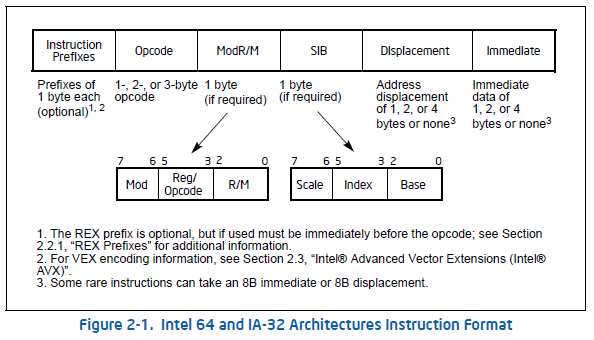# Instruction - ModR/M Byte

The ModR/M byte is a part of an instruction used when a memory operand is required (used).

It permit to specify:

## Syntax

Below is a picture of an intel instruction format (From appendix b instruction formats and encodings) where you can see the ModR/M field.

Certain encodings of the ModR/M byte require a second addressing byte (the SIB byte).

## Table

ModR/M byte has $2^8 = 256$ possible values by CPU word size (16, 32, … bit): seen under the label Value of ModR/M Byte (in Hexadecimal) in the tables called Addressing form table. There is actually one table by CPU Word Size (ie 16-bit or 32-bit)

Example with the table in 16-bit addressing forms (Section 2.1.5 - Page 509 of the Intel® 64 and IA-32 Architectures Software Developer’s Manual

The figure below demonstrates the interpretation of one ModR/M value. Example for C8.

ModR/M value       C8  = 11001000
----------------
Mod = 11
RM  =      000
/digit (Opcode) or REG =   001



### First operand

The Effective Address column lists 32 effective addresses and one of them is assigned to the first operand of an instruction.

The selection of an Effective Address is made by using the combination of:

• the Mod value
• and the R/M value

The Mod field, or modifier field combined with rm field gives a total of $5$ bits of information to specify (encode) $2^5 = 32$ possible values:

• 24 addressing modes. The first 24 lines. They provide ways of specifying a memory location)
• 8 registers. The last eight (Mod = 11B) are for each line a list of 5 register (general-purpose, MMX technology and XMM registers). The register selected is determined by the opcode byte along with the operand-size attribute.

For example: The row indicated by:

• Mod = 11,
• R/M = 000.

identifies multiple registers in the effective address column:

• the general-purpose registers EAX, AX or AL;
• MMX technology register MM0;
• or XMM register XMM0.

The register selected is determined by the opcode byte along with the operand-size attribute.

### Reg/Opcode

The Reg/Opcode field encodes either:

• a second register operand If an instruction requires it.
• or extends the Opcode field with 3 more bits. In cases where the reg/opcode field in the ModR/M byte represents an extended opcode, valid encodings are shown in Appendix B.

#### Second operand

If the instruction requires a second operand, the seventh row (labeled “REG =”) specifies the use of the 3-bit regopcode field when the field is used to give the location of a second operand that must be a register (general purpose, MMX technology, or XMM)

Rows one through five list the registers. The register selected is determined by:

#### Opcode extension

If the instruction does not require a second operand, then the regopcode field may be used as an opcode extension. This use is represented by the sixth row in the tables (labeled “/digit (Opcode)”). Note that values in row six are represented in decimal form.

## disp

• The disp16 nomenclature denotes a 16-bit displacement that follows the ModR/M byte and that is added to the index.
• The disp8 nomenclature denotes an 8-bit displacement that follows the ModR/M byte and that is sign-extended and added to the index.

Recommended PagesIntel Instruction Interpretation

This section is based on the section 3-1 - interpreting the instruction pages reference of the intel documentation and used the jmp mnemonic as example. Definition: The jmp mnemonic transfers program...